# Any straight way to know the start month number as per Current FY?Hi Friends, is there any formula / straight way to know the month number of the Current Fiscal Year? e.g. if the CURRENT FISCAL YEAR in Time Setting is as below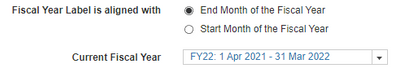Then Apr'2021 is first month of FY22. How to calculate like below?

TIME SCALE --> Apr  May  Jun  Jul ...... Mar

LINE ITEM -->     1       2       3      4  ...... 12

Tagged:

•Create a time module with line items and formula as per below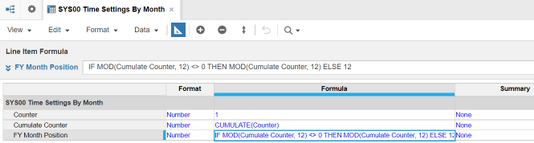It should result in the following: (I've hidden some months so you can see the results across years)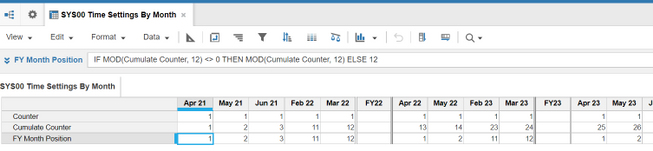•Try this

Step 1) Create a Time Calculation module as below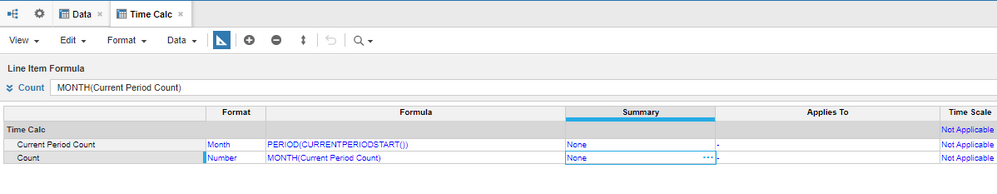write the formulas as written above.

Step 2) Create the data module with time as a dimension as shown below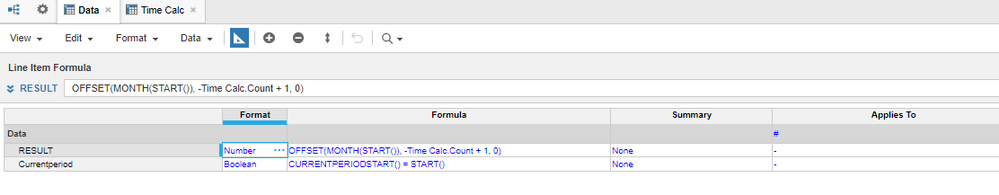Your result should look like below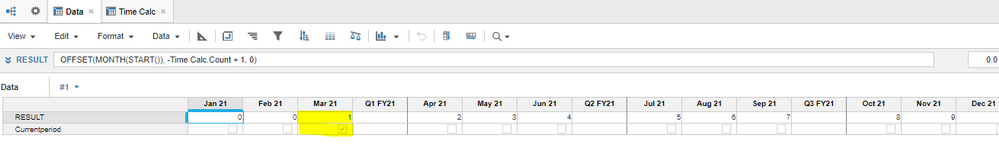~Anand